Latest Banking jobs   »   Quantitative Aptitude Quiz For IBPS PO...

# Quantitative Aptitude Quiz For IBPS PO Mains 2022-13th January

Directions (1-5): In the given questions, two quantities are given, one as Quantity I and another as Quantity II. You have to determine relationship between two quantities and choose the appropriate option

Q1. In a two digit number, digit at unit place exceeds, the digit in its tens place by 2 and the product of the required number with the sum of its digit is equal to 144.
Quantity I: Value of two digit number
Quantity II: 26
(a) Quantity I > Quantity II
(b) Quantity I < Quantity II
(c) Quantity I ≥ Quantity II
(d) Quantity I ≤ Quantity II
(e) Quantity I = Quantity II or No relation

Q2. Quantity I : Days after which A and B meet. A and B set out to meet each other from two places 165 km apart. A travels 15 km the first day, 14 km second day, 13 km the third day and so on, B travels 10 km the first, 12 km the second day, 14 km the third day and so on.
Quantity II: Number of days required to complete the whole work if A, B and C can complete a piece of work in 10, 12 and 15 days respectively. A left the work 5 days before the work was completed and B left 2 days after A had left.
(a) Quantity I > Quantity II
(b) Quantity I < Quantity II
(c) Quantity I ≥ Quantity II
(d) Quantity I ≤ Quantity II
(e) Quantity I = Quantity II or No relation

Q3. Quantity I: Present age of Randy, if 10 years are subtracted from the present age of Randy, then you would get twelve times of the present age of his grandson Sandy and Sandy is 19 years younger to Sundar whose age is 24.
Quantity II: Average age of the remaining persons in the group if average age of group of 14 persons is 27 years and 9 months. Two persons, each 42 years old, left the group.
(a) Quantity I > Quantity II
(b) Quantity I < Quantity II
(c) Quantity I ≥ Quantity II
(d) Quantity I ≤ Quantity II
(e) Quantity I = Quantity II or No relation

Q4. Quantity I: Percentage profit earned by the shopkeeper if at the time of selling and purchasing he uses weights 10% less and 20% more per kilogram respectively and proffesses to all goods at 5% profit.
Quantity II: ‘x’ ; A book was sold for a certain sum and there was a loss of 20%. Had it been sold for Rs 12 more, there would have been a gain of 30%. ‘x’ would be value of profit percent if the book were sold for Rs 4.8 more than what it was sold for.
(a) Quantity I > Quantity II
(b) Quantity I < Quantity II
(c) Quantity I ≥ Quantity II
(d) Quantity I ≤ Quantity II
(e) Quantity I = Quantity II or No relation

Q5. A group consist of 4 couples in which each of the 4 persons have one wife
Quantity I : Number of ways in which they could be arranged in a straight line such that the men and women occupy alternate positions
Quantity II: Eight times the number of ways in which they be seated around circular table such that men and women occupy alternate position.
(a) Quantity I > Quantity II
(b) Quantity I < Quantity II
(c) Quantity I ≥ Quantity II
(d) Quantity I ≤ Quantity II
(e) Quantity I = Quantity II or No relation

Directions (6-10): The following questions are accompanied by three statements A, B and C. You have to determine which statement(s) is/are necessary/sufficient to answer the question.

Q6. How many times does the efficiency of a man exceed that of a woman?
A. 3 women and 2 men working together complete a work in 6 days.
B. 3 men do the same work 5 days sooner than 9 women.
C. Ratio of the efficiencies of 8 women and 4 men is 1 : 3.
(a) Any two of the three together
(b) All the three together
(c) Only C
(d) Only A and B together
(e) Either C alone or A and B together.

Q7. Find out the length of train A.
A. Train A crosses another train B moving in same direction in 72 sec.
B. Speed of train A is 25km/h more than that of train B.
C. Length of train B is 20% less than that of train A.
(a) All the three together are not sufficient
(b) Only A and C together
(c) All the three together
(d) Only A and B together
(e) Only B and C together

Q8. Find lateral surface area of a cylinder.
A. Volume of a cone with base same as that of the cylinder and height 30 cm is equal to volume of the cylinder.
B. Circumference of base of the cylinder is 132 cm.
C. Volume of the cylinder is 13860 cm3.
(a) Only A and B together
(b) Only A and C together
(c) All the three together
(d) Any two of the three together
(e) Either A and B together or A and C together

Q9. There are some red, blue and green balls in bag. What is the probability of getting a red ball if one ball is selected at random?
A. Ratio of number of green and blue balls in the bag is 4 : 3.
B. If we add 2 more red balls in the bag, the numbers of red balls will be equal to the number of green balls.
C. Sum of number of green and blue balls is twice the number of red balls in the bag.
(a) C alone
(b) Either C alone or A and B together
(c) Any two of the three together
(d) Only A and B together
(e) All the three together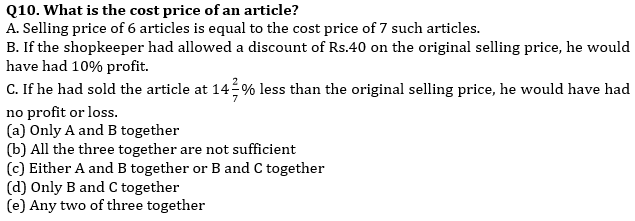Solutions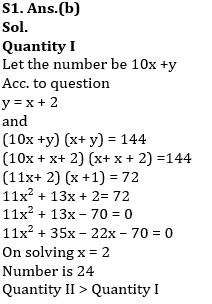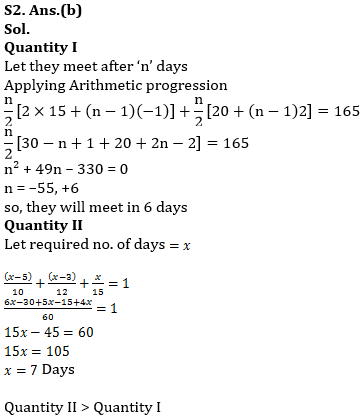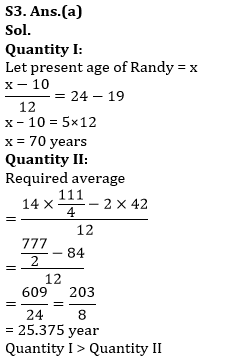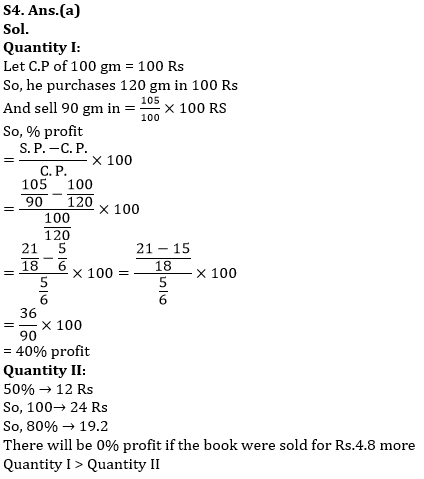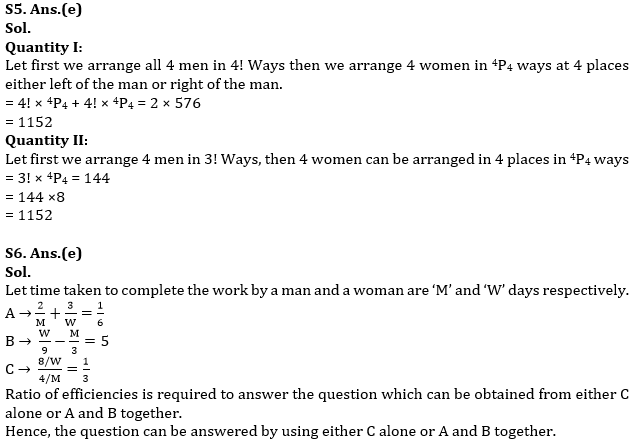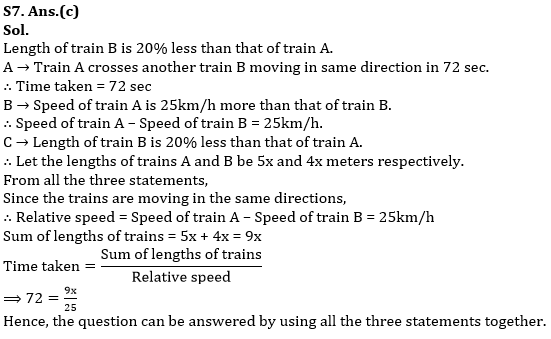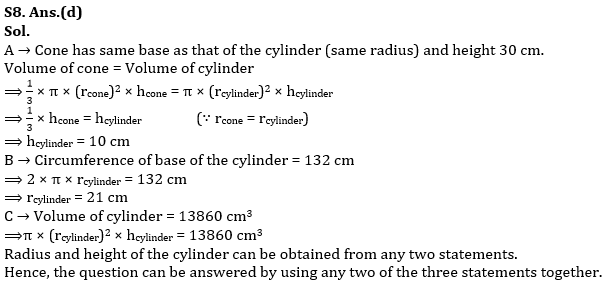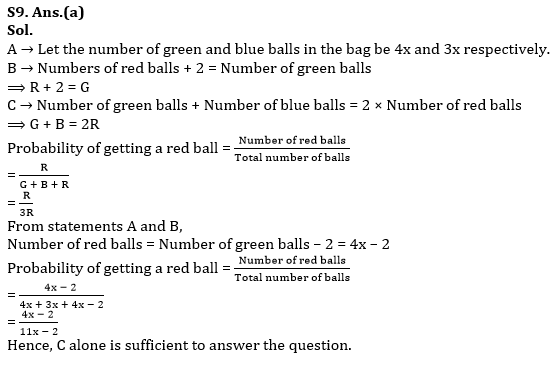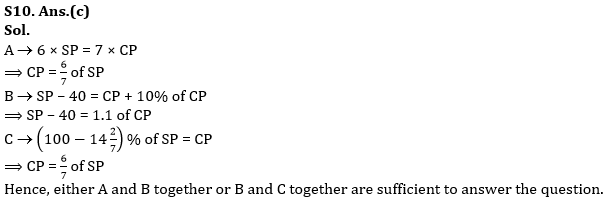#### Congratulations!Union Budget 2023-24: Free PDF